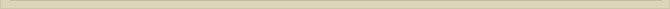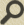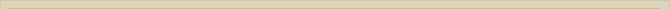## The stellar aberration and dragged ether

When you are interested in physics you must read “Unbelievable“!

A photon can be accredited an impulse in the direction of movement equal to: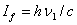Impulse of the photon in the X- and Y- direction in ether II for the observer in rest with ether II is (figure 2):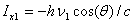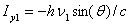Because ether II moves always sideways with speed v respectively to ether I, the impulse of the photon for the observer in rest with ether I in the X-direction, when the movement is maximal v towards the star (figure 4 and 5 the movement in Y-direction is zero), will be: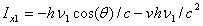(Bradley only measured the stellar aberration in the X-direction; the vertical aberration. When the movement towards the star is zero {speed in X-direction is zero and the movement in the Y-direction is therefore v} the horizontal aberration, not measured Bradley is maximal)

In the Y-direction the impulse of the photon is not changed, because the speed of both ether to each other in that direction is zero:For the observer in ether II the impulse of the photon, after penetrating ether II from ether I, will not change. The vacuum has not the quality to change the impulse. Considering this the stellar aberration can now be determined the following equations: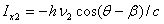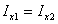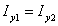The reduced formulas are: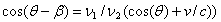The stellar aberration and the Doppler-effect in the X-direction, due to the passage of the photon from ether I to ether II, is described the equations: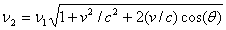These formulas only describe the stellar aberration and Doppler-effect caused the speed in X-direction towards or from the star (vertical aberration). Bradley did not measure the horizontal aberration caused the speed of the Earth in the Y-direction; sideways to the star. The vector summation of the vertical aberration caused the speed of the Earth in the X-direction and the horizontal aberration caused the speed of the Earth in the Y-direction gives the total aberration of the star y-Draconis.

Considering these formulas we observe a small difference when we calculate ß for v and the reverse speed –v. This small deviation between both calculations of the aberration β for v and -v  is caused the math that does not describe the actual physics exactly. The summation of both β for v and -v should be exactly zero. The formulas above describe the situation where the speed changes instantaneous from zero to v or –v. In reality the speed gradually increases from zero to v and than gradually to –v. This second order effect, not described the formulas, is responsible for the slight difference between the calculated ß with v or –v.

Next chapterThe drag coefficient of Fresnel